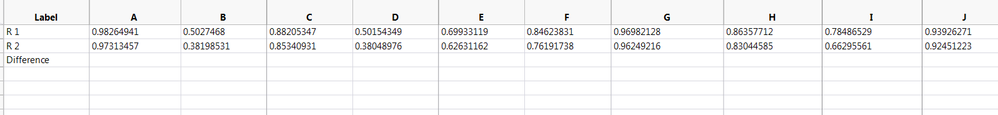Choose Language Hide Translation Bar
Highlighted

## How can i subtract row values

How can I subtract values of R1 from R2 and add the answer to the Difference row?3 REPLIES 3
Highlighted

## Re: How can i subtract row values

``````dt = Current Data Table();

Mat = dt << Get As Matrix;

Res = Mat[1,0] - Mat[2,0];

MatFinal = V Concat(Mat,Res);

dt_New = As Table(MatFinal);``````
Best
Uday
Highlighted

## Re: How can i subtract row values

Here is a simple script that will do what you are asking

``````Names Default To Here( 1 );

// Create a sample data table
dt = New Table( "Untitled 4",
New Column( "Label",
Character,
"Nominal",
Set Values( {"#1", "#2", "Difference"} )
),
New Column( "A",
Numeric,
"Continuous",
Format( "Best", 12 ),
Set Values( [5, 6, .] ),
Set Display Width( 43 )
),
New Column( "B",
Numeric,
"Continuous",
Format( "Best", 12 ),
Set Values( [9, 7, .] )
),
New Column( "C",
Numeric,
"Continuous",
Format( "Best", 12 ),
Set Values( [12, 4, .] ),
Set Display Width( 43 )
)
);

// Here is the code to subtract the values
For( i = 2, i <= N Cols( dt ), i++,
Column( dt, i ) = Column( dt, i ) - Column( dt, i )
);``````
Jim
Highlighted

## Re: How can i subtract row values

The key to what you are asking is to remember that a JMP data table is NOT a spreadsheet.  What I would suggest is to transpose your data table and then add a new column of differences.  Then you are set to analyze the difference by the groups that you currently have in columns.

1. Table > Transpose

2. Select your label column as the "Label" and select all other columns and click "Transposne Columns" and click ok.

Now  you have a new table with a column of labels for your "groups" (what were your columns) and two colums of data, one for R1 and one for R2.

3. From this new table higlight the two R columns, right click, select New Formula Column > Combine > Difference.

Now you have column of differences and you can analyze how the differences differ across your groups (i.e., use graph builder with the group lables as the x and the differences as the y).

Article Labels

There are no labels assigned to this post.